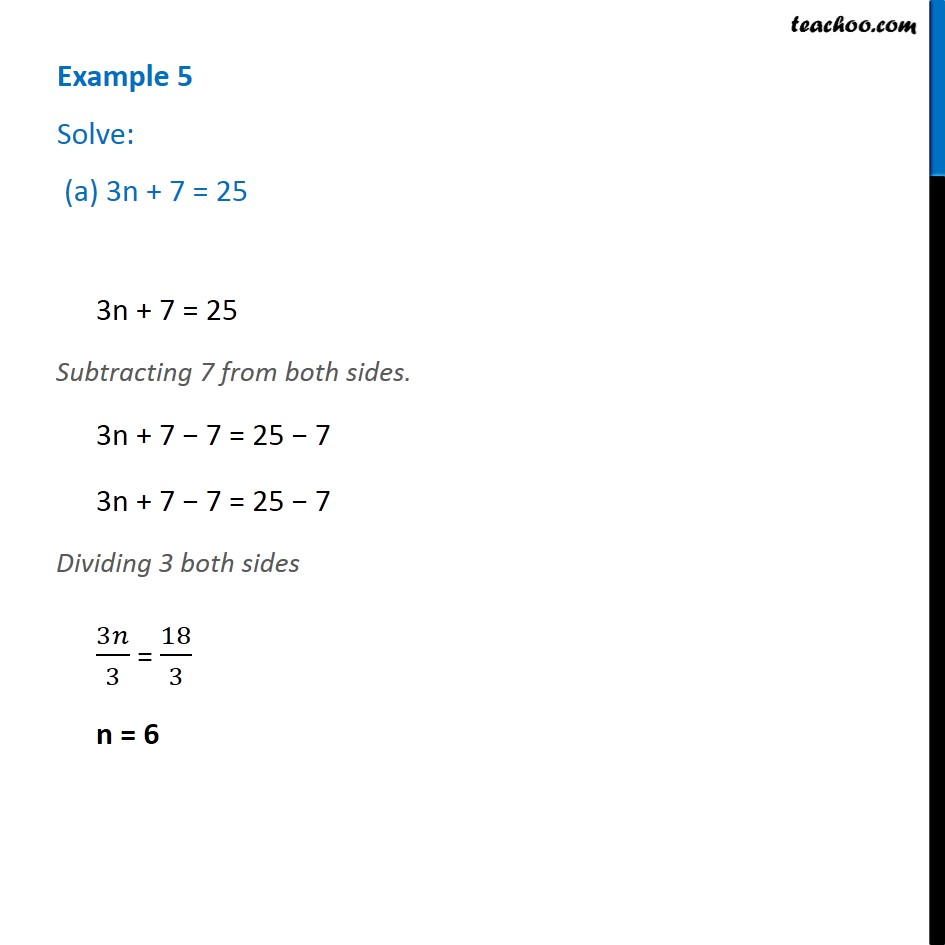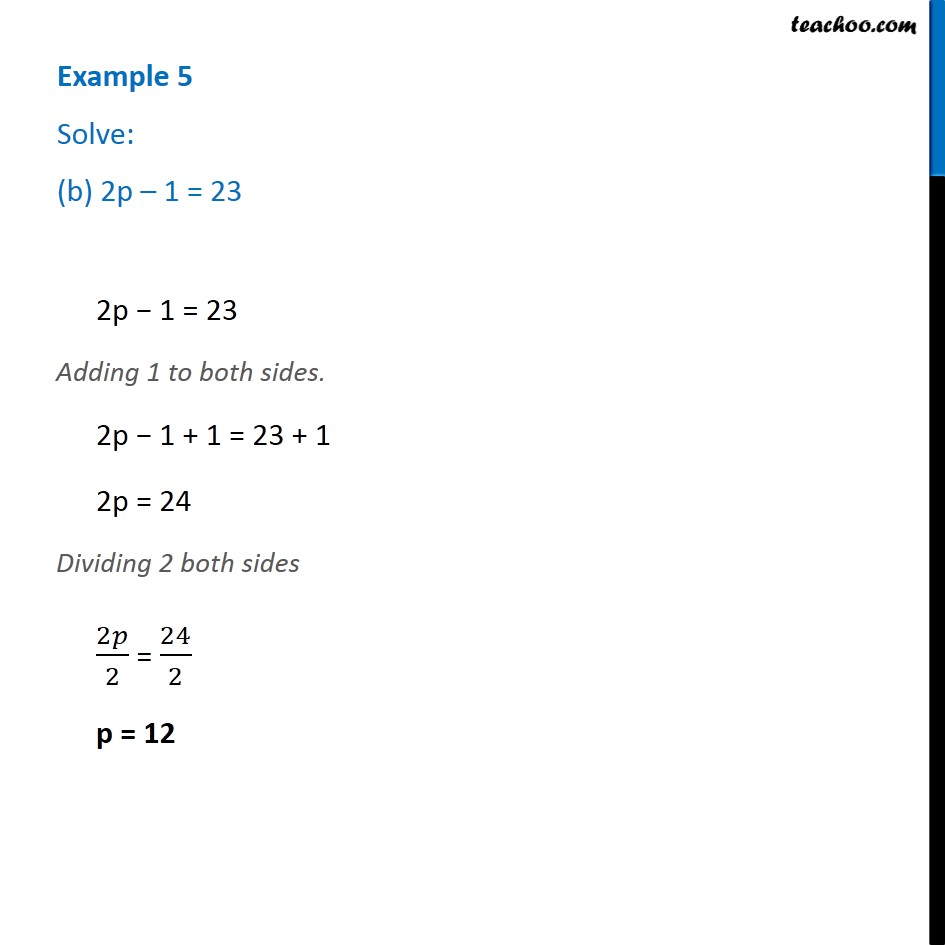Subscribe to our Youtube Channel - https://you.tube/teachoo

1. Chapter 4 Class 7 Simple Equations
2. Serial order wise
3. Examples

Transcript

Example 5 Solve: (a) 3n + 7 = 253n + 7 = 25 Subtracting 7 from both sides. 3n + 7 − 7 = 25 − 7 3n + 7 − 7 = 25 − 7 Dividing 3 both sides 3𝑛/3 = 18/3 n = 6 3n + 7 = 25 Subtracting 7 from both sides. 3n + 7 − 7 = 25 − 7 3n + 7 − 7 = 25 − 7 Dividing 3 both sides 3𝑛/3 = 18/3 n = 6 Example 5 Solve: (b) 2p – 1 = 23 2p − 1 = 23 Adding 1 to both sides. 2p − 1 + 1 = 23 + 1 2p = 24 Dividing 2 both sides 2𝑝/2 = 24/2 p = 12

Examples